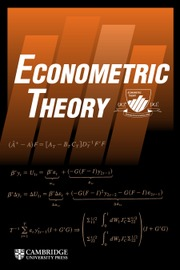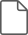Home
Hostname: page-component-7f7b94f6bd-2h7tr Total loading time: 0.406 Render date: 2022-06-28T12:48:15.217Z Has data issue: true Feature Flags: { "shouldUseShareProductTool": true, "shouldUseHypothesis": true, "isUnsiloEnabled": true, "useRatesEcommerce": false, "useNewApi": true } hasContentIssue trueEconometric Theory

# ADAPTIVE NONPARAMETRIC REGRESSION WITH CONDITIONAL HETEROSKEDASTICITY

Published online by Cambridge University Press:  08 September 2014

*
*Address correspondence to Sainan Jin, School of Economics, Singapore Management University, 90 Stamford Road, Singapore 178903; e-mail: snjin@smu.edu.sg.

## Abstract

In this paper, we study adaptive nonparametric regression estimation in the presence of conditional heteroskedastic error terms. We demonstrate that both the conditional mean and conditional variance functions in a nonparametric regression model can be estimated adaptively based on the local profile likelihood principle. Both the one-step Newton–Raphson estimator and the local profile likelihood estimator are investigated. We show that the proposed estimators are asymptotically equivalent to the infeasible local likelihood estimators [e.g., Aerts and Claeskens (1997) Journal of the American Statistical Association 92, 1536–1545], which require knowledge of the error distribution. Simulation evidence suggests that when the distribution of the error term is different from Gaussian, the adaptive estimators of both conditional mean and variance can often achieve significant efficiency over the conventional local polynomial estimators.

Type
ARTICLES
Information
Econometric Theory , December 2015 , pp. 1153 - 1191

## Access options

Get access to the full version of this content by using one of the access options below. (Log in options will check for institutional or personal access. Content may require purchase if you do not have access.)

## References

Aerts, M. & Claeskens, G. (1997) Local polynomial estimation in multiparameter likelihood models. Journal of the American Statistical Association 92, 15361545.CrossRefGoogle Scholar
Ai, C. (1997) A semiparametric maximum likelihood estimator. Econometrica 65, 933963.CrossRefGoogle Scholar
Akritas, M. & Van Keilegom, I. (2001) Nonparametric estimation of the residual distribution. Scandinavian Journal of Statistics 28, 549568.CrossRefGoogle Scholar
Andrews, D.W.K. (1994a) Asymptotics for semiparametric econometric models via stochastic equicontinuity. Econometrica 62, 4372.CrossRefGoogle Scholar
Andrews, D.W.K. (1994b) Empirical process methods in econometrics. In Engle, R.F. & McFadden, D. (eds.), Handbook of Econometrics, Vol. IV. North-Holland.Google Scholar
Andrews, D.W.K. (1995) Nonparametric kernel estimation for semiparametric models. Econometric Theory 11, 560596.CrossRefGoogle Scholar
Bernstein, D.S. (2005) Matrix Mathematics: Theory, Facts, and Formulas with Application to Linear Systems Theory. Princeton University Press.Google Scholar
Bickel, P.J. (1982) On adaptive estimation. Annals of Statistics 10, 647671.CrossRefGoogle Scholar
Claeskens, G. & Aerts, M. (2000) On local estimating equations in additive multiparameter models. Statistics and Probability Letters 49, 139148.CrossRefGoogle Scholar
Claeskens, G. & Van Keilegom, I. (2003) Bootstrap confidence bands for regression curves and their derivatives. Annals of Statistics 31, 18521884.Google Scholar
Fan, J. (1992) Design-adaptive nonparametric regression. Journal of the American Statistical Association 87, 9981004.CrossRefGoogle Scholar
Fan, J. (1993) Local linear regression smoothers and their minimax efficiencies. Annals of Statistics 21, 196216.CrossRefGoogle Scholar
Fan, J., Farmen, M., & Gijbels, I. (1998) Local maximum likelihood estimation and inference. Journal of the Royal Statistics Society, Series B 60, 591608.CrossRefGoogle Scholar
Fan, J. & Gijbels, I. (1996) Local Polynomial Modelling and Its Applications. Chapman and Hall.Google Scholar
Fan, J., Heckman, N.E., & Wand, M.P. (1995) Local polynomial kernel regression for generalized linear models and quasi-likelihood functions. Journal of the American Statistical Association 90, 141150.CrossRefGoogle Scholar
Fan, J. & Yao, Q. (1998) Efficient estimation of conditional variance functions in stochastic regression. Biometrika 85, 645660.CrossRefGoogle Scholar
Hansen, B.E. (2008) Uniform convergence rates for kernel regression. Econometric Theory 24, 726748.CrossRefGoogle Scholar
Härdle, W. & Tsybakov, A. (1997) Local polynomial estimators of the volatility function in nonparametric autoregression. Journal of Econometrics 81, 233242.CrossRefGoogle Scholar
Kreiss, J. (1987) On adaptive estimation in stationary ARMA process. Annals of Statistics 15, 112133.CrossRefGoogle Scholar
Lee, A.J. (1990) U-statistics: Theory and Practice. Marcel Dekker.Google Scholar
Linton, O. & Xiao, Z. (2007) A nonparametric regression estimator that adapts to error distribution of unknown form. Econometric Theory 23, 371413.CrossRefGoogle Scholar
Masry, E. (1996a) Multivariate regression estimation: Local polynomial fitting for time series. Stochastic Processes and Their Applications 65, 81101.CrossRefGoogle Scholar
Masry, E. (1996b) Multivariate local polynomial regression for time series: Uniform strong consistency rates. Journal of Time Series Analysis 17, 571599.CrossRefGoogle Scholar
Rudin, W. (1976) Principles of Mathematical Analysis, 3rd ed. McGraw-Hill.Google Scholar
Ruppert, D., Wand, M.P., Holst, U., & Hössjer, O. (1997) Local polynomial variance function estimation. Technometrics 39, 262273.CrossRefGoogle Scholar
Staniswalis, J.E. (1989) On the kernel estimate of a regression function in likelihood-based models. Journal of the American Statistical Association 84, 276283.CrossRefGoogle Scholar
White, H. (1994) Estimation, Inference and Specification Analysis. Cambridge University Press.CrossRefGoogle Scholar
Ziegelmann, F. (2002) Nonparametric estimation of volatility functions: The local exponential estimator. Econometric Theory 18, 985991.CrossRefGoogle Scholar### Jin et al. supplementary material

Supplementary data

File 135 KB
4
Cited by

# Save article to Kindle

Note you can select to save to either the @free.kindle.com or @kindle.com variations. ‘@free.kindle.com’ emails are free but can only be saved to your device when it is connected to wi-fi. ‘@kindle.com’ emails can be delivered even when you are not connected to wi-fi, but note that service fees apply.

Find out more about the Kindle Personal Document Service.

ADAPTIVE NONPARAMETRIC REGRESSION WITH CONDITIONAL HETEROSKEDASTICITY
Available formats
×

# Save article to Dropbox

To save this article to your Dropbox account, please select one or more formats and confirm that you agree to abide by our usage policies. If this is the first time you used this feature, you will be asked to authorise Cambridge Core to connect with your Dropbox account. Find out more about saving content to Dropbox.

ADAPTIVE NONPARAMETRIC REGRESSION WITH CONDITIONAL HETEROSKEDASTICITY
Available formats
×

# Save article to Google Drive

To save this article to your Google Drive account, please select one or more formats and confirm that you agree to abide by our usage policies. If this is the first time you used this feature, you will be asked to authorise Cambridge Core to connect with your Google Drive account. Find out more about saving content to Google Drive.

ADAPTIVE NONPARAMETRIC REGRESSION WITH CONDITIONAL HETEROSKEDASTICITY
Available formats
×
×